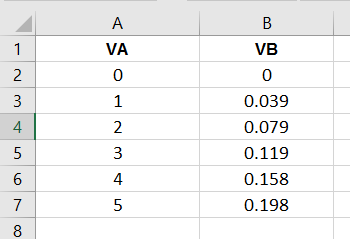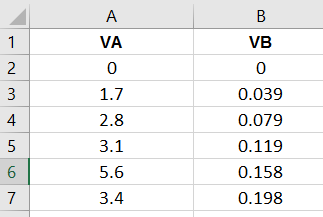# How to get Below and Above value of entered value from JSON list?

Hello Everyone,

How can we get the Below and Above values of entered value?

I have below JSON list

[
{
“A”: “1”,
“B”: “K1”
},
{
“A”: “2”,
“B”: “K2”
},
{
“A”: “3”,
“B”: “K3”
},
{
“A”: “4”,
“B”: “K4”
}
]

If the user entered Value A = 2.4, Then How can I get the available Below and Above value from this list.

Example
Entered Value A = 2.4
Then
Below Value of A from the list is = 2 &
Above Value of A from the list is = 3

and also How Can I get an Index of Below & Above Values?

Will your json have this stucture 1,2,3,… etc ? What will happen if value is 2.9 below value again will be 2 and above 3? What will happen if number entered is not in list ?

This is a procedure to get data from above json

1 Like

I have data in excel like belowI converted it into JSON like below

``````[
{
"VA": "0",
"VB": "0"
},
{
"VA": "1",
"VB": "0.039"
},
{
"VA": "2",
"VB": "0.079"
},
{
"VA": "3",
"VB": "0.119"
},
{
"VA": "4",
"VB": "0.158"
},
{
"VA": "5",
"VB": "0.198"
}
]
``````

I need to build logic for

Logic No.1
If the user entered VA = 2
Then Result need to come= 0.079 (This Value is VB)

Logic No. 2
If the user entered VA = 2.9 (Note: This value is not available in the sheet, but we need to find out the nearest lower value of 2.9 which is 2 & nearest higher value of 2.9 which is 3)

If I achieved to get lower and higher values then I have a formula to get the value of VB

First case check if number entered in textbox is integer, then if true check if is in list VA, and if true then select item in list VB index number’s index in list VA

If number is not integer, split at . and get first item in list, check it that number is in list VA, check if that number plus 1 is in list VA, then set lower value to that number and higher to number +1

Thanks for this idea.

Please note that I have some data like below then I am unable to do as @dora_paz said. Because All VA values are not an integer. and also every time VA value not increasing +1
Then How Can I do that.Hello @dora_paz,
Have any updates regarding my 2nd logic?